• +91 9971497814
• info@interviewmaterial.com

# RD Chapter 8- Lines and Angles Ex-8.3 Interview Questions Answers

### Related Subjects

Question 1 : In figure, lines l1, and l2 intersect at O, formingangles as shown in the figure. If x = 45. Find the values of y, z and u.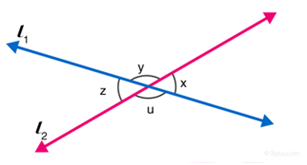Given: x = 450

Since vertically opposite angles are equal, therefore z = x = 450

z and u are angles that are a linear pair, therefore, z + u =1800

Solve, z + u = 1800 , for u

u = 1800 – z

u = 1800 – 45

u = 1350

Again, x and y angles are a linear pair.

x+ y = 1800

y = 1800 – x

y =1800 – 450

y = 1350

Hence, remaining angles are y = 1350, u = 1350 andz = 450.

Question 2 :

In figure, threecoplanar lines intersect at a point O, forming angles as shown in the figure.Find the values of x, y, z and u .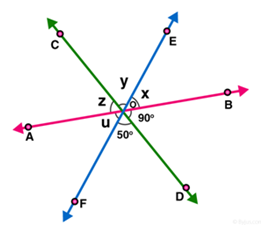(BOD,z); (DOF, y) are pair of vertically opposite angles.

So, BOD = z= 900

DOF = y= 500

[Vertically oppositeangles are equal.]

Now, x + y + z = 180 [Linear pair] [AB is a straight line]

x + y + z = 180

x + 50 + 90 = 180

x = 180 – 140

x = 40

Hence values of x, y, z and u are 400, 500,900 and 400 respectively.

Question 3 : In figure, find the values of x, y and z.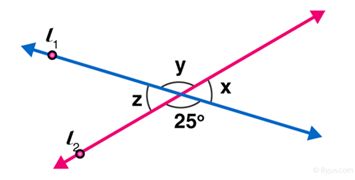From figure,

y = 250 [Vertically opposite angles are equal]

Now x + y = 1800 [Linear pair ofangles]

x = 180 – 25

x = 155

Also, z = x = 155 [Vertically opposite angles]

Answer: y = 250 and z = 1550

Question 4 :

In figure, find thevalue of x.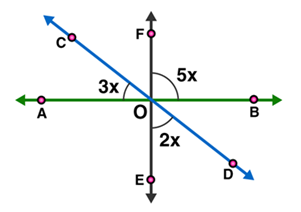AOE = BOF = 5x [Vertically opposite angles]

COA+AOE+EOD = 1800 [Linear pair]

3x + 5x + 2x = 180

10x = 180

x = 180/10

x = 18

The value of x = 180

Question 5 :

Prove thatbisectors of a pair of vertically opposite angles are in the same straightline.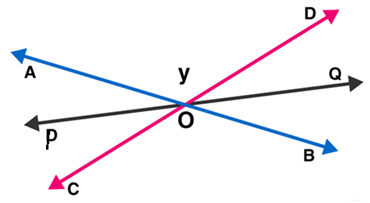Lines AB and CD intersect at point O, such that

AOC = BOD (vertically angles) …(1)

Also OP is the bisector of AOC and OQ is the bisector of BOD

To Prove: POQ is a straight line.

OP is the bisector of AOC:

AOP = COP …(2)

OQ is the bisector of BOD:

BOQ = QOD …(3)

Now,

Sum of the angles around a point is 360o.

AOC + BOD + AOP + COP + BOQ + QOD = 3600

BOQ + QOD + DOA + AOP + POC + COB = 3600

2QOD + 2DOA + 2AOP = 3600 (Using (1), (2) and (3))

QOD + DOA + AOP = 1800

POQ = 1800

Which shows that, the bisectors of pair of vertically oppositeangles are on the same straight line.

Hence Proved.

Question 6 :

If two straightlines intersect each other, prove that the ray opposite to the bisector of oneof the angles thus formed bisects the vertically opposite angle.

Given AB and CD are straight lines which intersect at O.

OP is the bisector of  AOC.

To Prove : OQ is the bisector of BOD

Proof :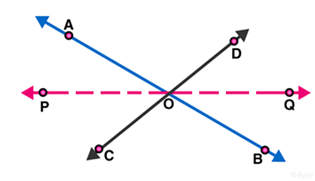AB, CD and PQ are straight lines which intersect in O.

Vertically opposite angles: AOP =  BOQ

Vertically opposite angles: COP =  DOQ

OP is the bisector of  AOC :  AOP=  COP

Therefore,  BOQ =  DOQ

Hence, OQ is the bisector of BOD.

Todays Deals### RD Chapter 8- Lines and Angles Ex-8.3 Contributorskrishan

Name:
Email:

# Latest News# 9000 interview questions in different categories